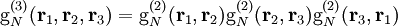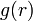Kirkwood superposition approximation${\rm g}_N^{(3)}({\mathbf r}_1,{\mathbf r}_2,{\mathbf r}_3)={\rm g}_N^{(2)}({\mathbf r}_1,{\mathbf r}_2){\rm g}_N^{(2)}({\mathbf r}_2,{\mathbf r}_3){\rm g}_N^{(2)}({\mathbf r}_3,{\mathbf r}_1)$
It appears that this was used as a basis of a closure for the Kirkwood integral equation (Ref. 1) and the Yvon, and Born-Green (Ref. 2) until the work of Morita and Hiroike (Ref. 3). It was pointed out in Ref.s 4 and 5, that there is an inconsistency between the pressure and the compressibility equation if this superposition approximation is used to generate$g(r)$. This approximation is rigorously correct for one-dimensional systems, and is only true in three-dimensions in the limit of zero density. It has recently been shown that the Kirkwood superposition approximation precludes the existence of a critical point (Ref. 6).